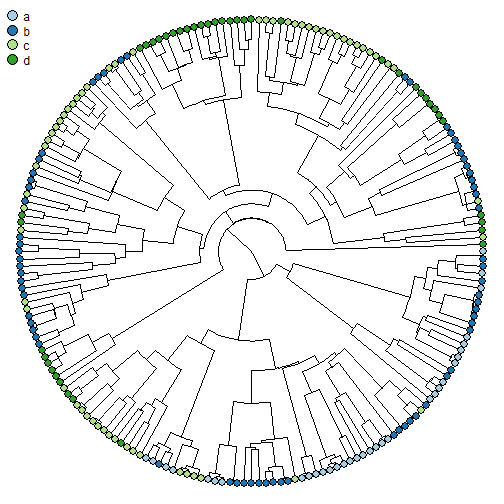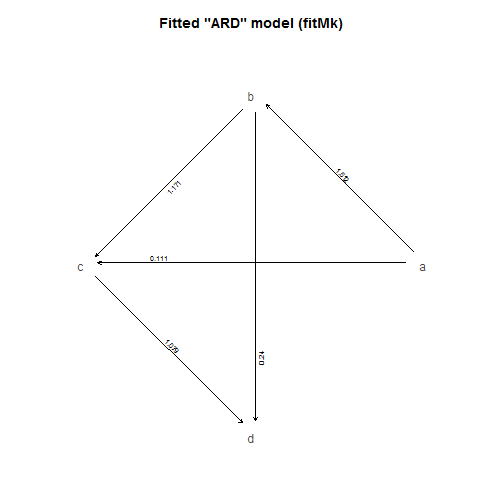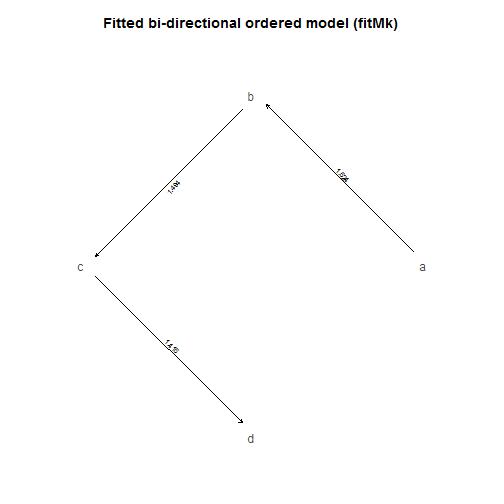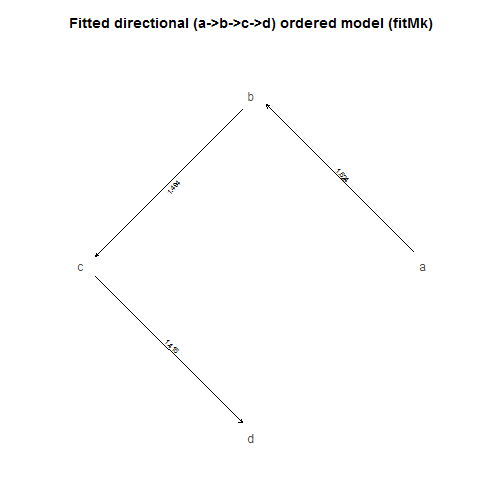## Friday, November 17, 2017

### Small but important update to `fitMk` and `make.simmap`

I just pushed a small update (1, 2) that will nonetheless be important if using the phytools function `fitMk` (for, unsurprisingly, fitting the Mk discrete character evolution model & its variants) or `make.simmap` under the default condition.

This update is inspired by reports that `make.simmap` has been running very slowly since the latest CRAN update of phytools.

The update eliminates the phytools function `expm` which was exported to the namespace - a function that wrapped `msm::MatrixExp` that in turn wrapped `expm::expm` - making me concerned that part of the reason the function was running slow might have been some kind of namespace conflict between `phytools::expm` & `expm::expm`. The new internal (and thus not exported) function is called `EXPM` and also checks to to see if the matrix is symmetric (using `base::isSymmetric`). If symmetric, `EXPM` uses `ape::matexpo` - which is faster than `expm::expm`, but inaccurate if the matrix to be exponentiated is not symmetric.

Here is a simple test with some simulated data:

``````library(phytools)
packageVersion("phytools")
``````
``````##  '0.6.45'
``````
``````library(RColorBrewer)
plotTree(tree,type="fan",lwd=1,ftype="off")
tiplabels(pie=to.matrix(x,levels(x)),
piecol=brewer.pal(length(levels(x)),"Paired"),
cex=0.4)
legend(x=par()\$usr,y=par()\$usr,legend=levels(x),
pt.cex=2,pch=21,pt.bg=brewer.pal(length(levels(x)),
"Paired"),bty="n")
``````I'll start by fitting the `"ARD"` or all-rates-different model:

``````fitARD<-fitMk(tree,x,model="ARD")
plot(fitARD,show.zeros=F)
title(main="Fitted \"ARD\" model (fitMk)")
````````````fitARD
``````
``````## Object of class "fitMk".
##
## Fitted (or set) value of Q:
##           a         b         c        d
## a -1.623692  1.512465  0.111227 0.000000
## b  0.000000 -1.410860  1.170768 0.240092
## c  0.000000  0.000000 -1.078893 1.078893
## d  0.000000  0.000000  0.000000 0.000000
##
## Fitted (or set) value of pi:
##    a    b    c    d
## 0.25 0.25 0.25 0.25
##
## Log-likelihood: -128.460484
##
## Optimization method used was "nlminb"
``````

Note that this is similar, but not precisely equal, to what is obtained from `geiger::fitDiscrete`. I previously showed this to be due to different assumptions about the prior at the root node of the tree:

``````fit.geiger<-fitDiscrete(tree,x,model="ARD")
fit.geiger
``````
``````## GEIGER-fitted comparative model of discrete data
##  fitted Q matrix:
##                   a             b             c             d
##     a -1.623684e+00  1.512461e+00  1.112234e-01  5.161506e-18
##     b  3.974284e-18 -1.410860e+00  1.170773e+00  2.400874e-01
##     c  5.154285e-16  7.782172e-16 -1.078897e+00  1.078897e+00
##     d  5.838474e-16  2.827941e-16  5.113579e-14 -5.200243e-14
##
##  model summary:
##  log-likelihood = -127.074190
##  AIC = 278.148380
##  AICc = 279.816829
##  free parameters = 12
##
## Convergence diagnostics:
##  optimization iterations = 100
##  failed iterations = 0
##  frequency of best fit = 0.08
##
##  object summary:
##  'lik' -- likelihood function
##  'bnd' -- bounds for likelihood search
##  'res' -- optimization iteration summary
##  'opt' -- maximum likelihood parameter estimates
``````

`fitMk` uses a 'flat' prior for the root, although the function also allows the user to specify an arbitrary prior. `fitDiscrete` uses a prior that I'm sure is statistically well-justified, but is not flat.

Luckily, `fitDiscrete` also outputs a likelihood function for the model - which we can actually re-optimize using any value for the prior that we choose. Below, I show that so-doing results & forcing a flat prior results in identical estimated rate parameters to `fitMk`:

``````lik<-fit.geiger\$lik
fit.flat<-optim(rep(1,length(fitARD\$rates)),lik,root="flat",
method="L-BFGS-B",lower=rep(1e-12,
length(fitARD\$rates)),upper=rep(Inf,length(fitARD\$rates)),
control=list(fnscale=-1))
fit.flat
``````
``````## \$par
##   1.512453e+00 1.112249e-01 1.000000e-12 1.000000e-12 1.170781e+00
##   2.400849e-01 1.000000e-12 1.000000e-12 1.078885e+00 1.000000e-12
##  1.000000e-12 1.000000e-12
##
## \$value
##  -128.4605
##
## \$counts
##       18       18
##
## \$convergence
##  0
##
## \$message
##  "CONVERGENCE: REL_REDUCTION_OF_F <= FACTR*EPSMCH"
``````
``````## estimated transition matrix
Qhat<-matrix(NA,length(fitARD\$states),length(fitARD\$states))
Qhat[]<-c(0,fit.flat\$par)[t(fitARD\$index.matrix) + 1]
diag(Qhat)<-0
diag(Qhat)<--rowSums(Qhat)
colnames(Qhat)<-rownames(Qhat)<-fitARD\$states
round(Qhat,6)
``````
``````##           a         b         c        d
## a -1.623678  1.512453  0.111225 0.000000
## b  0.000000 -1.410866  1.170781 0.240085
## c  0.000000  0.000000 -1.078885 1.078885
## d  0.000000  0.000000  0.000000 0.000000
``````

OK, let's also fit a ordered & a directional model to compare:

``````## ordered bi-directional model
ordered<-matrix(c(0,1,0,0,
2,0,3,0,
0,4,0,5,
0,0,6,0),4,4,byrow=TRUE)
ordered
``````
``````##      [,1] [,2] [,3] [,4]
## [1,]    0    1    0    0
## [2,]    2    0    3    0
## [3,]    0    4    0    5
## [4,]    0    0    6    0
``````
``````fitOrdered<-fitMk(tree,x,model=ordered)
fitOrdered
``````
``````## Object of class "fitMk".
##
## Fitted (or set) value of Q:
##           a         b         c        d
## a -1.624262  1.624262  0.000000 0.000000
## b  0.000000 -1.493648  1.493648 0.000000
## c  0.000000  0.000000 -1.414502 1.414502
## d  0.000000  0.000000  0.000000 0.000000
##
## Fitted (or set) value of pi:
##    a    b    c    d
## 0.25 0.25 0.25 0.25
##
## Log-likelihood: -129.687402
##
## Optimization method used was "nlminb"
``````
``````plot(fitOrdered,show.zeros=FALSE)
title(main="Fitted bi-directional ordered model (fitMk)")
````````````## ordered directional model (a->b->c->d)
directional<-matrix(c(0,1,0,0,
0,0,2,0,
0,0,0,3,
0,0,0,0),4,4,byrow=TRUE)
directional
``````
``````##      [,1] [,2] [,3] [,4]
## [1,]    0    1    0    0
## [2,]    0    0    2    0
## [3,]    0    0    0    3
## [4,]    0    0    0    0
``````
``````fitDirectional<-fitMk(tree,x,model=directional)
fitDirectional
``````
``````## Object of class "fitMk".
##
## Fitted (or set) value of Q:
##           a         b         c        d
## a -1.624262  1.624262  0.000000 0.000000
## b  0.000000 -1.493649  1.493649 0.000000
## c  0.000000  0.000000 -1.414502 1.414502
## d  0.000000  0.000000  0.000000 0.000000
##
## Fitted (or set) value of pi:
##    a    b    c    d
## 0.25 0.25 0.25 0.25
##
## Log-likelihood: -129.687402
##
## Optimization method used was "nlminb"
``````
``````plot(fitDirectional,show.zeros=FALSE)
title(main="Fitted directional (a->b->c->d) ordered model (fitMk)")
``````It's pretty easy to compare among these models & see that our 'directional' model is best justified by the data:

``````aic<-setNames(c(AIC(fitDirectional),AIC(fitOrdered),
AIC(fitARD)),c("directional","ordered","ARD"))
aic
``````
``````## directional     ordered         ARD
##    265.3748    271.3748    280.9210
``````
``````aic.w(aic) ## weights
``````
``````## directional     ordered         ARD
##  0.95219234  0.04740687  0.00040079
``````

That's good - because that's exactly what I simulated, as follows:

``````tree<-pbtree(n=200,scale=1)
tol<-1e-12
Q<-matrix(c(-1-2*tol,1,tol,tol,
tol,-1.5-2*tol,1.5,tol,
tol,tol,-2,2,
tol,tol,tol,-3*tol),4,4,byrow=TRUE)
rownames(Q)<-colnames(Q)<-letters[1:4]
x<-as.factor(sim.history(tree,t(Q),anc="a")\$states)
``````

Cool.